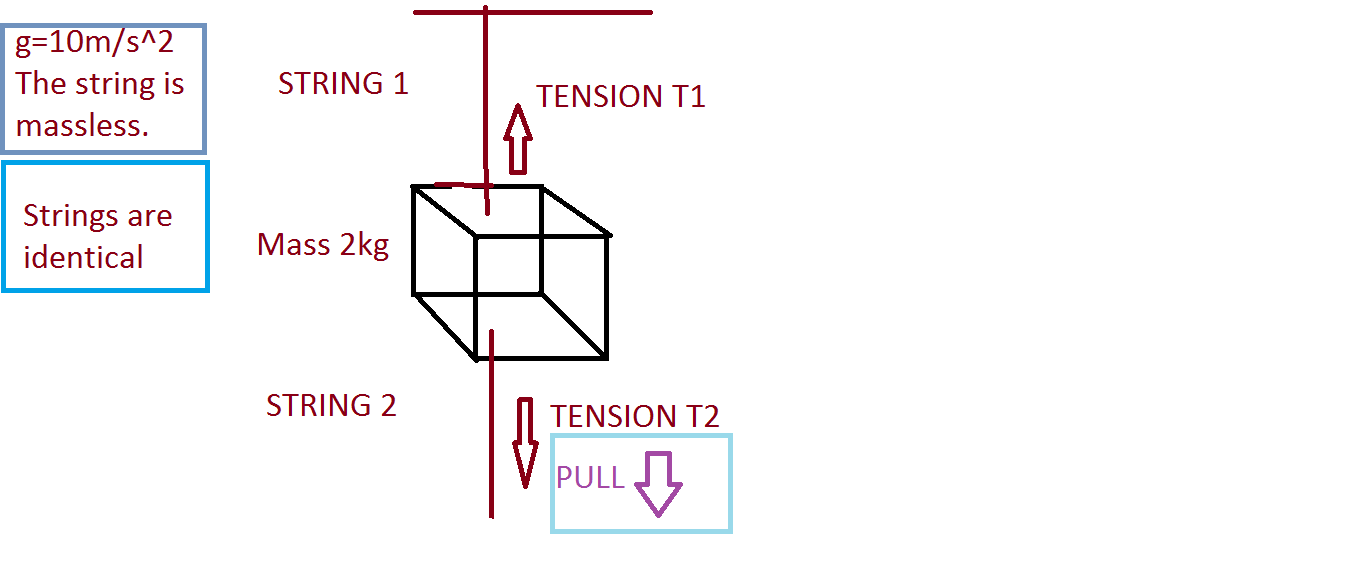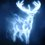# DECOY WEIGHT PROBLEMIn the given figure, a pull is applied in the downward direction so our intuition says that the string 1 must break first.It is because initially T1=20 N(weight of block) and T2=0(mass of string is assumed 0).So, the top string must break, nevertheless at MIT an experiment was carried out a few years ago , where in the first case the bottom string broke and the next time it was done ,the top one broke.PLEASE NOTE THAT THIS WAS NOT A PART OF ANY MAGIC SHOW. Here is my explanation for this : Case 1: I put in a pull on the bottom string which initially lied perpendicular the surface.The top string held an object of weight 20 N.Now since our strings our identical, the top string exerts a greater force than the bottom one, so the top string must break at first hand ,but it does not.There is some hidden force which is either increasing T2 or decreasing T1 by the same factor such that it becomes equal.It is quite obvious that this hidden force is gravity which is pulling the block downwards counteracting the tension T1 and thus keeping the whole system at 0 acceleration. Therefore ,the tension T1 became equal to T2 . But as I applied a pull downwards the tension T2 suddenly increased, and it broke. Case 2: Initially tension T1=20 N, T2=0 N.As I pulled ,the tension T1 increased because now it's magnitude was 20N + my pull. During the pull, the block changed it's state of rest and by Newton's first law, a body can change it's state of motion only when applied by an external unbalanced force.So the block accelerated (forget the magnitude).The string 1 now had to pull even harder so as to keep the block from falling.Therefore it broke. Any one can ask that why in this case 'the tension T2 not increase suddenly'? It is because ,that it did increase but only with the value of my pull., while the T1 was way beyond this .One can also say that why didn't in the first case the system experiance a downward acceleration? It is because in the first case the system did not have any time to readjust itself the sudden increase from 0 up to my pull caused string to break.

I do not think the whole of this thing is correct.There is some fallacy in this . If the readers find some better explanation then it is expected to be posted.Note by Raven Herd
7 years, 2 months ago

This discussion board is a place to discuss our Daily Challenges and the math and science related to those challenges. Explanations are more than just a solution — they should explain the steps and thinking strategies that you used to obtain the solution. Comments should further the discussion of math and science.

When posting on Brilliant:

• Use the emojis to react to an explanation, whether you're congratulating a job well done , or just really confused .
• Ask specific questions about the challenge or the steps in somebody's explanation. Well-posed questions can add a lot to the discussion, but posting "I don't understand!" doesn't help anyone.
• Try to contribute something new to the discussion, whether it is an extension, generalization or other idea related to the challenge.
• Stay on topic — we're all here to learn more about math and science, not to hear about your favorite get-rich-quick scheme or current world events.

MarkdownAppears as
*italics* or _italics_ italics
**bold** or __bold__ bold
- bulleted- list
• bulleted
• list
1. numbered2. list
1. numbered
2. list
Note: you must add a full line of space before and after lists for them to show up correctly
paragraph 1paragraph 2

paragraph 1

paragraph 2

[example link](https://brilliant.org)example link
> This is a quote
This is a quote
    # I indented these lines
# 4 spaces, and now they show
# up as a code block.

print "hello world"
# I indented these lines
# 4 spaces, and now they show
# up as a code block.

print "hello world"
MathAppears as
Remember to wrap math in $$ ... $$ or $ ... $ to ensure proper formatting.
2 \times 3 $2 \times 3$
2^{34} $2^{34}$
a_{i-1} $a_{i-1}$
\frac{2}{3} $\frac{2}{3}$
\sqrt{2} $\sqrt{2}$
\sum_{i=1}^3 $\sum_{i=1}^3$
\sin \theta $\sin \theta$
\boxed{123} $\boxed{123}$

## Comments

There are no comments in this discussion.

×

Problem Loading...

Note Loading...

Set Loading...